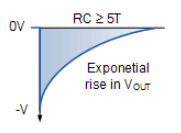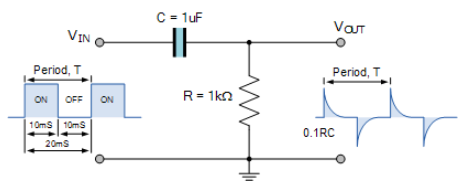# RC 微分器### RC 微分电路## 电阻器电压### 电容器电流

$$\mathrm { i } _ { ( \mathrm { t } ) } = \frac { \mathrm { d } \mathrm { Q } } { \mathrm { dt } } = \frac { \mathrm { d } \left( \mathrm { C } \times \mathrm { d } \mathrm { V } _ { \mathrm { C } } \right) } { \mathrm { dt } } = \mathrm { C } \frac { \mathrm { d } \mathrm { V } _ { \mathrm { C } } } { \mathrm { dt } } = \mathrm { C } \frac { \mathrm { d } \mathrm { V } _ { \mathrm { IN } } } { \mathrm { dt } }$$

$$\mathrm { i } _ { \mathrm { C } ( \mathrm { t } ) } = \mathrm { C } \frac { \mathrm { dV } _ { \mathrm { IN } ( \mathrm { t } ) } } { \mathrm { dt } }$$

$$\begin{array} { l } { \mathrm { V } _ { \mathrm { OUT } } = \mathrm { V } _ { \mathrm { R } } = \mathrm { R } \times \mathrm { i } _ { \mathrm { R } } } \\ { \mathrm { i } _ { \mathrm { C } } = \mathrm { C } \frac { \mathrm { d } \mathrm { V } _ { \mathrm { IN } } } { \mathrm { dt } } } \end{array}$$

$$\mathrm { V } _ { \mathrm { OUT } } = \mathrm { R } \mathrm { C } \frac { \mathrm { d } V _ { \mathrm { IN } } } { \mathrm { dt } }$$

### RC 微分公式

$$V _ { O U T } = R C \frac { d V _ { I N } } { d t }$$

## 单脉冲 RC 微分器### RC 微分器输出波形### RC 微分器示例